# Grade - math word problems

#### Number of problems found: 5415

• Unknown number 6Determine the unknown number, which is by 1.5 greater than its fourth.
• Rectangle areaThe length of a rectangle of x units is increased by 10% and its width of y units is increased by 15%. What is the ratio of the area of the old rectangle to the area if the new rectangle?
• TrapeziumThe area of trapezium is 35 cm2. Find its altitude if the bases are 6cm and 8 cm.
• Square prismCalculate the volume of a square prism of high 2 dm wherein the base is: rectangle with sides 17 cm and 1.3 dm
• Average speed 4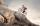Starting at home Tony traveled uphill to the store for 45 minutes at 8 miles per hour. he then traveled back home on the same path at a speed of 24 miles per hour. what is his average speed for the entire trip?
• Assembly parts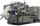Nine machines produce 1,800 parts on nine machines. How many hours will it produce 2 100 parts on seven such machines?
• Tulips and daffodils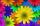Farm cultivated tulips and 211 units on 50 units more daffodils. How many spring flowers grown together?
• One threeWe throw two dice. What is the probability that max one three falls?
• The ticketsThe tickets to the show cost some integer number greater than 1. Also, the sum of the price of the children's and adult tickets, as well as their product, was the power of the prime number. Find all possible ticket prices.
• Two trainsThe train runs at speed v1 = 72 km/h. The passenger, sitting in the train, observed that a train long l = 75m in 3 s passed on the other track in the opposite direction. Calculate the speed of this train.
• Progression-12, 60, -300,1500 need next 2 numbers of pattern
• Mixed2improperWrite the mixed number as an improper fraction. 166 2/3
• Lee isLee is 8 years more than twice Park's age, 4 years ago, Lee was three times as old. How old was Lee 4 years ago?
• Length subtractingExpress in mm: 5 3/10 cm - 2/5 mm
• Car parking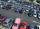The car park has 80 cars when 53 cars leaves. How many car are there?
• Cuboid easyThe cuboid has the dimensions a = 12 cm, b = 9 cm, c = 36 cm. Calculate the length of the body diagonal of the cuboid.
• Right triangle - ratioThe lengths of the legs of the right triangle ABC are in ratio b = 2: 3. The hypotenuse is 10 cm long. Calculate the lengths of the legs of that triangle.
• TreesA young tree is 16 inches tall. One year later, it is 20 inches tall. What is the percent increase in height?
• Two circles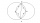Two circles with the same radius r = 1 are given. The center of the second circle lies on the circumference of the first. What is the area of a square inscribed in the intersection of given circles?
• Waste paper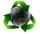Our school attend 1,300 pupils. Each pupil collected plastic bottles or paper. Plastic bottles have collected 930 pupils and 600 pupils old paper. How many students have collected together plastic bottles and waste paper?

Do you have an interesting mathematical word problem that you can't solve it? Submit a math problem, and we can try to solve it.

We will send a solution to your e-mail address. Solved examples are also published here. Please enter the e-mail correctly and check whether you don't have a full mailbox.

Please do not submit problems from current active competitions such as Mathematical Olympiad, correspondence seminars etc...